# Subdividing a Mixed String into Separate Cells Containing only Numbers or Characters in Microsoft Excel 2010

In this article we will learn how to subdivide a mixed string into separate cells which contain only numbers or characters,we can use the "TRIM", "LEFT", "FIND", and "SUBSTITUTE" functions in Microsoft Excel 2010.

FIND:This function returns the location number of the character at which a specific character or text string is first found, reading left to right (not case-sensitive).

Syntax of “FIND” function: =FIND

(find_text,within_text,[start_num])

Example:Cell A1 contains the text “Broncho Billy Anderson”

=FIND ("Billy", A1, 1), function will return 9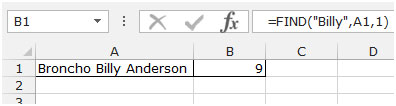LEFT: Returns the specified number of characters starting from the left-most character in the string.

Syntax of “LEFT” function: =LEFT (text,[num_chars])

Example:Cell A1contains the text “Broncho Billy Anderson”

=LEFT (A1, 7), function will return “Broncho”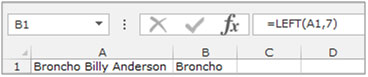TRIM: Trim function is used to remove additional spaces from the text in a cell.

Syntax of “TRIM” function: =TRIM (text)

Example: Cell A2 contains the text.

• Write the text in cell B2
• =TRIM(A2), press Enter on your keyboard
• The function will return the text after deleting the unnecessary spaces.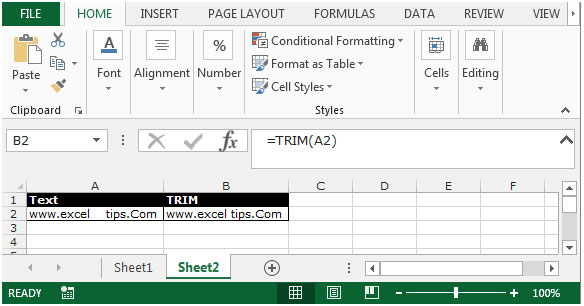SUBSTITUTE:This function is used to replace old text with new text in a cell.

Syntax of “SUBSTITUTE” function:

=SUBSTITUTE (text,old_text,new_text,[instance_num])

Example: Cell A2 contains the text “Petrol”

=SUBSTITUTE (A2, A2,"Diesel")

The function will returnDiesel.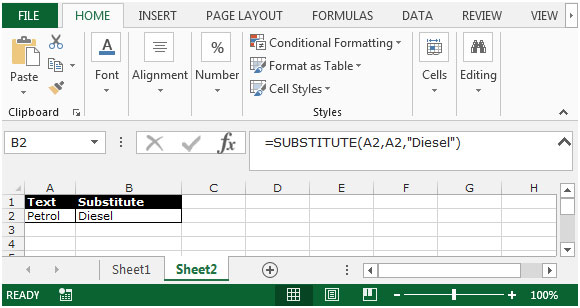Let’s take an example to understand how we can subdivide a mixed string into separate cells containing only numbers or characters.

Example- The strings in column Aare composed of both numbers and characters. However the numbers appear at the beginning of the string and the characters at the end.

We need to separate the strings into two columns, one containing only numbers and the other containing the characters.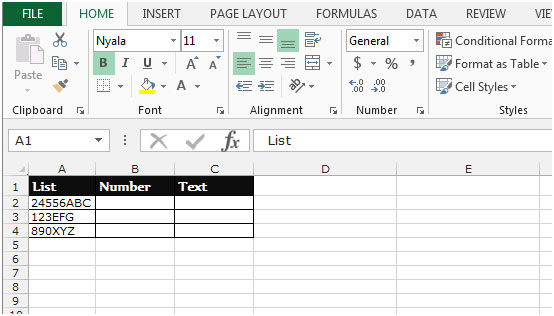• Select the cell B2 and write the formula
• =LEFT(A2,MAX(IF(ISNUMBER(-MID(A2,ROW(INDIRECT("1:1024")),1)),ROW(INDIRECT("1:1024")))))
• The function willreturn the number in cell B2.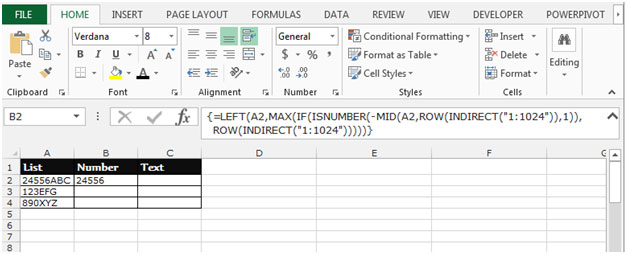• Copy the formula in all cells, press the key “CTRL + C” and select the cells B3:B4 and press the key “CTRL + V” on your keyboard.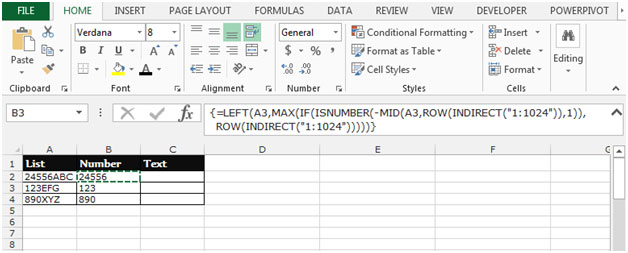To return only the text from cell A2, use the Substitute function as shown in the following formula entered in column C:-

• Write the formula in cell C2
• =SUBSTITUTE(A2,B2,"")
• Press Enter on your keyboard.
• The function will return only the text from the cell A2.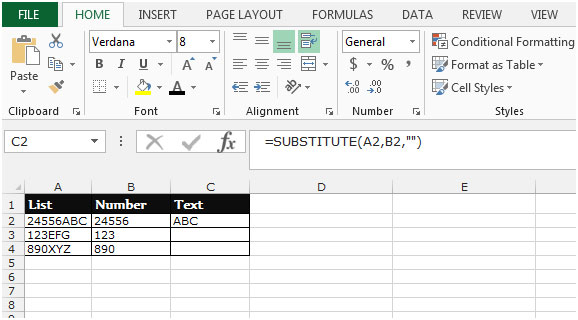• Copy the formula in all cells, press the key “CTRL + C” and select the cells C3:C4 and press the key “CTRL + V” on your keyboard.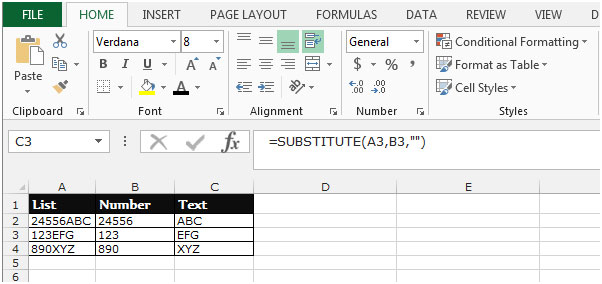This is howwe can subdivide a mixed string of numbers and characters into separate cells containing only numbers or characters in Microsoft Excel.

1.Hi! Maybe you can help me out. I have a string of numbers that I need to have individually listed, one number per cell. Right now that string is being identified by have the smallest number in one cell and the largest in another. Instead of displaying like this I need to have the entire string of numbers to display. What is a formula that I can use to do this? Thanks in advance!

2.•=LEFT(A2,MAX(IF(ISNUMBER(-MID(A2,ROW(INDIRECT(“1:1024?)),1)),ROW(INDIRECT(“1:1024?)))))

What is this 1:1024 in this formula? it is giving formula error....

•Hi Danish,

You are following wrong formula

Correct Formula is:- =LEFT(A2,MAX(IF(ISNUMBER(-MID(A2,ROW(INDIRECT("1:1024")),1)),ROW(INDIRECT("1:1024")))))

Not this •=LEFT(A2,MAX(IF(ISNUMBER(-MID(A2,ROW(INDIRECT(“1:1024?)),1)),ROW(INDIRECT(“1:1024?)))))